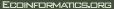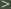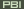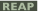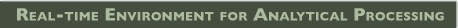# Ocean_SST_Usecase 4

Difference between version 3 and version 2:

 Line 1 was replaced by line 1 - Use Case #4 - ANALYZE Match-up Data Set + !!Usecase #4 - ANALYZE Match-up Data Set Lines 3-55 were replaced by lines 3-49 - Version - 1.0 - - Summary - - Obtain target minus comparison SST data set statistics from the match-up data base - - The interface should provide flexible access to a range of analytical capabilities. - - Input: A match-up data set generated by Use Case #3. - - Output: Statistical representations of the target minus comparison data set SST values. - - Preconditions - - Triggers - - Basic course of events - - 1) Define refinement parameters: - - nearest neighbor, - - average - - variance - - min-max difference - - 2) Refine match-up - - Calculate target, comparison SST pairs (1 SST value for each); e.g., using nearest neighbor, average, bi-linear interpolation,... - - Calculate statistic associated with target and comparison match-ups; e.g., variance, min-max difference, skewness,... - - 3) Define filter (or stratify) parameters: - - space - - time - - refined statistic - - target-comparison distance in space and time - - comparison data set - - 4) Define statistical analyses; - - histogram differences - - mean and variance - - 5) Apply statistical analyses on the refined match-up data set target minus comparison SST value - - 6) Output plots, tables - - Alternative paths - - Postconditions - - Business rules - - Notes - - Author and date - SST Use Case Development Group 25 July 2007. - + __Version:__ 1.0\\ + \\ + __Summary:__\\ + \\ + Obtain target minus comparison SST data set statistics from the match-up data base.\\ + \\ + The interface should provide flexible access to a range of analytical capabilities.\\ + \\ + *__Input:__ A match-up data set generated by Use Case #3. + *__Output:__ Statistical representations of the target minus comparison data set SST values. + \\ + __Preconditions:__\\ + \\ + __Triggers:__\\ + \\ + Basic course of events:\\ + + #Define refinement parameters: + - nearest neighbor,\\ + - average\\ + - variance\\ + - min-max difference\\ + #Refine match-up + - Calculate target, comparison SST pairs (1 SST value for each); e.g., using nearest neighbor, average, bi-linear interpolation,...\\ + - Calculate statistic associated with target and comparison match-ups; e.g., variance, min-max difference, skewness,...\\ + #Define filter (or stratify) parameters: + - space\\ + - time\\ + - refined statistic\\ + - target-comparison distance in space and time\\ + - comparison data set\\ + #Define statistical analyses; + - histogram differences\\ + - mean and variance\\ + #Apply statistical analyses on the refined match-up data set target minus comparison SST value + #Output plots, tables + + __Alternative paths:__\\ + \\ + __Postconditions:__\\ + \\ + __Business rules:__\\ + \\ + __Notes:__\\ + \\ + __Author and date -__ SST Use Case Development Group 25 July 2007.\\ + \\

Back to Ocean_SST_Usecase 4, or to the .